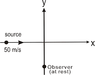# Frequency of sound received by an observer

PSN03
Homework Statement:
A source and an observer are situated on two perpendicular tracks as shown in the figure the observer is at rest and source is moving with a speed 50m/s the source emits sound waves of frequency 90Hz which travel in the medium with velocity 200m/s the frequency of sound heard by observer when the source crosses the origin is
Relevant Equations:
By dopler's equation we know that
f'=f(v±vo/v±vs)
Where
f'= new frequency
vo=velocity of observer
vs=velocity of source
v=velocity of sound
They are asking us to find the frequency of sound received by observer when the source reaches at origin. According to me when the source reaches origin there would be no component of source's velocity in the direction of observer, hence vs=0
vo=0 since the observer is stationary

Therefore by the formula I should get the answer as
f'=f(v±0/v±0)
=f
Hence no change in frequency should be observed. But my answer is wrong.
Lease tell me where am I going wrong?

#### Attachments

•1.8 KB · Views: 64

Homework Helper
How close is the observer to the crossing point of the tracks ?

Homework Helper
Gold Member
Hence no change in frequency should be observed. But my answer is wrong.
Lease tell me where am I going wrong?
It takes time for the sound to travel from the source to the observer. At the instant when the source crosses the origin, the observer is hearing the sound that was emitted by the source at an earlier time (when the source was not yet at the origin). Some geometry will come into play. So, you need a good sketch of the situation.

•PSN03 and etotheipi
PSN03
It takes time for the sound to travel from the source to the observer. At the instant when the source crosses the origin, the observer is hearing the sound that was emitted by the source at an earlier time (when the source was not yet at the origin). Some geometry will come into play. So, you need a good sketch of the situation.
Yes...I got it later. Thanks for your help and I feel so stupid for forgetting such a simple point.😅